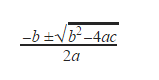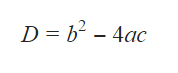# GMAT Quant: Algebra – Quadratic EquationsAny form of the equation having second-degree polynomial in one variable is called quadratic equation. Typically in GMAT you have to deal with the  equation form of y = ax2 + bx + c formula related to the quadratic equations that you need to mug up is

1. Root of the the equation ax2 + bx + c = 0 is1. Determinant D is calculated asIf D=0, equation will have real and equal root

If D>0, equation will have real roots

If D<0, equation will have imaginary roots

Let’s look at some problems

1. The sum of a number and its reciprocal is 10/3. Find the number.

Let the number be x.therefore x +1/x=10/3

Arranging the equation we get,

3x210x + 3 = 0

(3x-1)(x-3) = 0

Therefore, x = 3, ⅓

1. If x2 + bx + 72 = 0 has integral roots, find the number of values b can attain.

72 can be written as 2 x 2 x 2 x 3 x 3. Hence the number of positive pairs whose product is 72 is (3+1)(2+1)/2= 6.

Similarly, the number of negative pairs are 6. Hence 12 is the answer.

We’ll be glad to help you in your GMAT preparation journey. You can ask for any assistance related to GMAT and MBA from us by calling us at +918884544444. You can write to us at gmat@byjus.com.